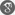##### Cite as:
A.A. Gaber, M.H. Shehata, New Approach of MHD Boundary Layer Flow Towards a Porous Stretching Sheet via Symmetry Analysis and the Generalized Exp-Function Method, Int. J. Anal. Appl., 18 (5) (2020), 738-747.

#### Abstract

Due to importance of the slip effect on modeling the boundary layer flows, symmetries and exact solution investigations have been introduced in this paper for studying the effect of a slip boundary layer on the stretching sheet through a porous medium. The exact solution of the investigating model is obtained in term of exponential via the generalized Exp-Function method. This solution satisfies the boundary conditions. Finally, the effect of parameters on the velocity field is studied.

#### References

1. L.V. Ovsiannikov, Group analysis of differential equations. Nauka, Moscow, 1978.2. P.J. Olver, Application of lie groups to differential equations. Springer-Verlag, 1986.3. N.K. Ibragimov, CRC handbook of Lie group analysis of differential equations, CRC Press, Boca Raton, 1994.4. G.W. Bluman, S. Kumei, Symmetries and Differential Equations, Springer-Verlag, New York, 1989.5. M.F. El-Sayed, G.M. Moatimid, M.H.M. Moussa, R.M. El-Shiekh, F.A.H. El-Shiekh, A.A. El-Satar, A study of integrability and symmetry for the (p + 1)th Boltzmann equation via Painlev´e analysis and Lie-group method, Math. Meth. Appl. Sci. 38 (2015), 3670–3677.6. M.H.M. Moussa, A.A. Gaber, Symmetry Analysis and Solitary Wave Solutions of Nonlinear Ion-Acoustic Waves Equation, Int. J. Anal. Appl. 18 (3) (2020), 448-460.7. M. Rosa, J.C. Camacho, M.S. Bruz´on, M.L. Gandarias, Classical and potential symmetries for a generalized Fisher equation, J. Comput. Appl. Math. 318 (2017), 181–188.8. J.C. Camacho, M. Rosa, M.L. Gandarias, M.S. Bruz´on, Classical symmetries, travelling wave solutions and conservation laws of a generalized Fornberg–Whitham equation, J. Comput. Appl. Math. 318 (2017), 149–155.9. R. Sinuvasan, K.M. Tamizhmani, P.G.L. Leach, Symmetries, travelling-wave and self-similar solutions of the Burgers hierarchy, Appl. Math. Comput. 303 (2017), 165–170.10. M. F. El-Sayed, G. M. Moatimid, M. H. M. Moussa, R. M. El-Shiekh and A. A. El-Satar, Similarity Reductions and New Exact Solutions for B-Family Equations, Amer. J. Math. Stat. 2 (3) (2012), 40-4311. B.C. Sakiadis, Boundary-layer behavior on continuous solid surfaces: I. Boundary-layer equations for two-dimensional and axisymmetric flow, AIChE J. 7 (1961), 26–28.12. M. Hatami, D.D. Ganji, Heat transfer and nanofluid flow in suction and blowing process between parallel disks in presence of variable magnetic field, J. Mol. Liquids. 190 (2014), 159–168.13. K. Bhattacharyya, S. Mukhopadhyay, G.C. Layek, I. Pop, Effects of thermal radiation on micropolar fluid flow and heat transfer over a porous shrinking sheet, Int. J. Heat Mass Transfer. 55 (2012), 2945–2952.14. A. Ebaid and Abdul-Majid Wazwaz, On the Generalized Exp-Function Method and Its Application to Boundary Layer Flow at Nano-Scale J. comput. theor. nanoscience, (2014), 11, 1–715. A.E.-H. Ebaid, Generalization of He’s Exp-Function Method and New Exact Solutions for Burgers Equation, Z. Naturforsch., A. 64 (2009), 604–608.16. E.M.E. Zayed, A.-G. Al-Nowehy, Exact solutions and optical soliton solutions for the (2 + 1)-dimensional hyperbolic nonlinear Schr¨odinger equation, Optik. 127 (2016), 4970–4983.